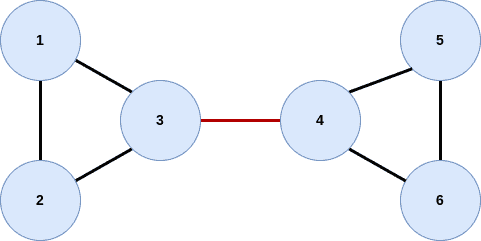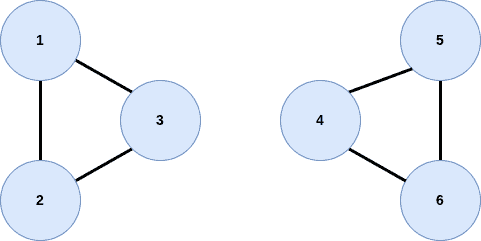## 1. Introduction

In computer science, a graph is a mathematical structure of objects that represent the relationships between them. Thus a graph includes nodes (objects) with edges (connections) that denote the relationships between them. Moreover, the edges of a graph can be directed or undirected.

In this tutorial, we’ll introduce the bridges in a graph, discuss their importance and discuss algorithms to detect them.

## 2. What Is a Bridge?

First of all, bridges, also known as cut edges, are specific types of edges in a graph. A bridge is a connection between two nodes that, if removed, causes the network to become unconnected and thus increases the number of linked nodes.

Suppose a graph, whereanddenote the vertices and edges, respectively. Therefore a bridge is defined as an edgeif no other path between the u and v nodes exists. So, if this edgeis removed from the graph, the vertices u and v will no longer be reachable from one another by any other path, and thus two sub-graphs are generated.

### 2.1. Different Types of Bridges

There are several kinds of bridges, each with its own characteristics. The most common ones are tree edges that belong in a tree graph structure. In this particular graph form, all links are bridges. Thus, removing one edge disconnects the whole graph. Moreover, there are the forward edges that belong to DFS trees. Forward edges link a node to one of its descendants in the DFS tree structure and, if removed, increase the number of connected components.

## 3. Example of a Bridge

Let’s consider the following undirected graph:As we can see, the edgeis a bridge because removing it disconnects the graph and creates two sub-graphs:The two sub-graphs include the nodes,,, and,,. Now there is no path from one sub-graph to the other, and the nodes,,cant be reached in any way from the nodes,,, and vice versa.

Note that if we removed, for example, the edge, paths would remain for every node to reach everyone else, and therefore we understand that the edgeisn’t a bridge in this graph.

## 4. Importance of a Bridges

A bridge indicates a crucial connection in a graph and offers useful insights and correlations about its structure. Cutting a bridge disconnects the graph, which can have a substantial influence on its overall connectivity. Furthermore, removing a bridge may separate a graph into two sections, which may be helpful for evaluating and comprehending the graph’s form. Also, the number of bridges in a graph is proportional to the number of linked components in the graph. The detection of bridges may be used in network design to increase the overall robustness and efficiency of a network by identifying and reinforcing essential links.

Moreover, bridges can be useful in various situations, such as determining the least spanning tree, identifying loops in a graph, and even addressing computational geometry issues.

Understanding bridges in a graph has the potential to have far-reaching significance in industries such as telecommunications, computer science, and transportation.

## 5. Algorithms for Finding Bridges

Several algorithms can be used to detect bridges in a graph. All of these algorithms show certain benefits and limitations and depend on the specific problem and the characteristics of the graph. The most common and straightforward algorithms are DFS (Depth-First Search) and BFS (Breadth-First Search), which are used to find all the articulation points or bridges in a graph.

DFS traverses the graph depth-first, keeps track of the low-link values of each vertex, and compares it with the values of the previously checked vertices. So if the low-link value of the current vertex is less than the low-link value of the adjacent vertex, then this edge is probably a bridge. DFS algorithm hastime complexity andspace complexity.

BFS follows a similar idea. It performs a breadth-first traversal of the graph, keeps track of the level of each vertex, and compares it with the level of the previously checked vertices. So, if the level of the current vertex is greater than the level of the adjacent vertex, then this edge is probably a bridge. BFS algorithm hastime complexity andspace complexity.

Another common method to detect bridges in a graph is Tarjan’s algorithm.

Note that it is not necessary for a graph to contain a bridge. However, large graphs of real-world networks usually contain a certain number of bridges to ensure the graph’s connectivity.

## 6. Conclusion

In this article, we introduced bridges in a graph. In particular, we talked about algorithms for finding bridges, showed a basic example of a bridge, and highlighted the importance of finding bridges in graphs.

Bridges help to understand the main structure of a graph along with its most significant components and are critical in analyzing the graph’s connectivity design and robustness.